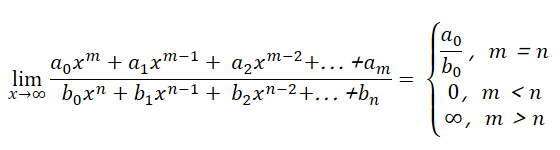# 极限运算法则

## Th3:

1)$\lim_{}[f(x)±g(x)]=\lim_{}f(x)±\lim_{}g(x)$

2)$\lim_{}[f(x)g(x)]=\lim_{}f(x) · \lim_{}g(x)$

3)如果$\lim_{}{\frac{f(x)}{g(x)}}=\frac{\lim_{}f(x)}{\lim_{}g(x)}$

## Th4：(极限的保序性)